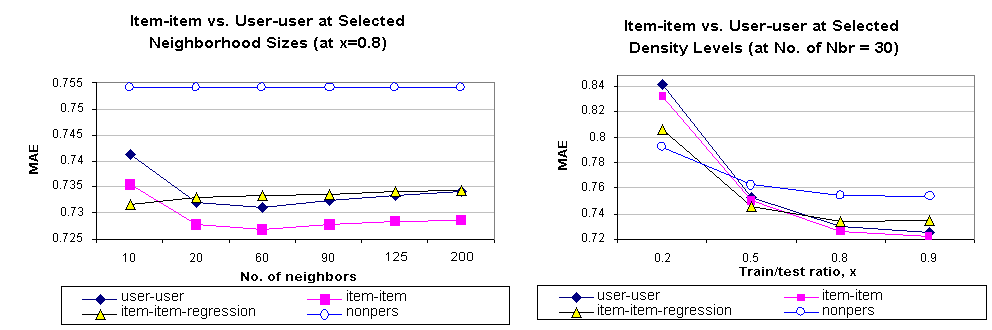Next: Performance Results Up: Experimental Results Previous: Experiments with neighborhood size

### Quality Experiments

Once we obtain the optimal values of the parameters, we compare both of our item-based approaches with the benchmark user-based algorithm. We present the results in Figure 6. It can be observed from the charts that the basic item-item algorithm outperforms the user based algorithm at all values of x(neighborhood size = 30 and all values of neighborhood size (x=0.8). For example, at x=0.5 user-user scheme has an MAE of 0.755 and item-item scheme shows an MAE of 0.749. Similarly at a neighborhood size of 60 user-user and item-item schemes show MAE of 0.732 and 0.726 respectively. The regression-based algorithm, however, shows interesting behavior. At low values of x and at low neighborhood size it out performs the other two algorithms but as the density of the data set is increased or as we add more neighbors it performs worse, even compared to the user-based algorithm. We also compared our algorithms against the naive nonpersonalized algorithm described in .

We draw two conclusions from these results. First, item-based algorithms provide better quality than the user-based algorithms at all sparsity levels. Second, regression-based algorithms perform better with very sparse data set, but as we add more data the quality goes down. We believe this happens as the regression model suffers from data overfiting at high density levels.Next: Performance Results Up: Experimental Results Previous: Experiments with neighborhood size